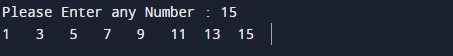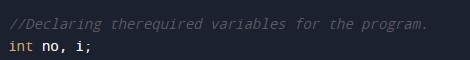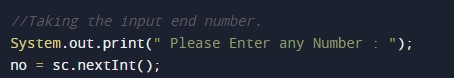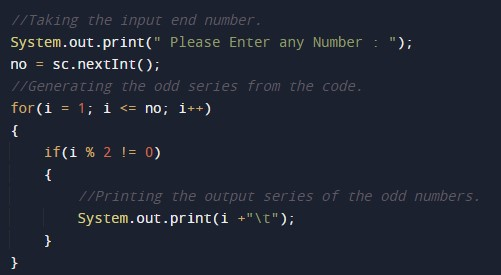# Odd Number Series Program in Java

In this tutorial you will learn about the Odd Number Series Program in Java and its application with practical example.

## Odd Number Series Program in Java

In this tutorial, we will learn to create a Java program that will Print Odd Number Series using Java programming.

## Prerequisites

Before starting with this tutorial, we assume that you are the best aware of the following Java programming topics:

• Operators in Java Programming.
• Basic Input and Output function in Java Programming.
• Basic Java programming.
• While loop in Java programming.
• Conditional Statements in Java programming.
• Arithmetic operations in Java Programming.

## What is an Odd number?

A number is said to be an Odd number when that number is divided by two into two equal whole numbers. And there should be a remainder of zero when it is being divided by 2.

## Program to Print Odd Numbers from 1 to N.

In this program, we will first take the input point from the user. Then we will find the Odd numbers up to that range. At last, we will print that series of Odd numbers up to that number.

With the help of this program, we can print a series of Odd numbers up to N number.

## Output:-In the above program, we have first initialized the required variable.• no = it will hold the integer value of the input.
• i = it will hold the integer value for the loop.

Input message for the user to take an endpoint of the series.Program Logic Code to generate the series of odd numbers.Printing output odd digitsIn this tutorial we have learn about the Odd Number Series Program in Java and its application with practical example. I hope you will like this tutorial.